# Python Audio

There are several ways to read and write audio files in Python, using different packages. This notebooks lists some options and discusses advantages as well as disadvantages. For detailed explanations on how to integrate audio files into the notebooks, we refer to the FMP notebook on Multimedia.

## LibROSA¶

One option to read audio is to use LibROSA's function librosa.load.

• Per default, librosa.load resamples the audio to $22050~\mathrm{Hz}$. Setting sr=None keeps the native sampling rate.
• The loaded audio is converted to a float with amplitude values lying in the range of $[-1, 1]$.
• librosa.load is essentially a wrapper that uses either PySoundFile or audioread.
• When reading audio, librosa.load first tries to use PySoundFile. This works for many formats, such as WAV, FLAC, and OGG. However, MP3 is not supported. When PySoundFile fails to read the audio file (e.g., for MP3), a warning is issued, and librosa.load falls back to another library called audioread. When ffmpeg is available, this library can read MP3 files.
In :
import os
import numpy as np
from matplotlib import pyplot as plt
import IPython.display as ipd
import librosa
import pandas as pd
%matplotlib inline

def print_plot_play(x, Fs, text=''):
"""1. Prints information about an audio singal, 2. plots the waveform, and 3. Creates player

Notebook: C1/B_PythonAudio.ipynb

Args:
x: Input signal
Fs: Sampling rate of x
text: Text to print
"""
print('%s Fs = %d, x.shape = %s, x.dtype = %s' % (text, Fs, x.shape, x.dtype))
plt.figure(figsize=(8, 2))
plt.plot(x, color='gray')
plt.xlim([0, x.shape])
plt.xlabel('Time (samples)')
plt.ylabel('Amplitude')
plt.tight_layout()
plt.show()
ipd.display(ipd.Audio(data=x, rate=Fs))

fn_wav = os.path.join('..', 'data', 'B', 'FMP_B_Note-C4_Piano.wav')
print_plot_play(x=x, Fs=Fs, text='WAV file: ')

fn_mp3 = os.path.join('..', 'data', 'B', 'FMP_B_Note-C4_Piano.mp3')
print_plot_play(x=x, Fs=Fs, text='MP3 file: ')

WAV file:  Fs = 11025, x.shape = (45504,), x.dtype = float32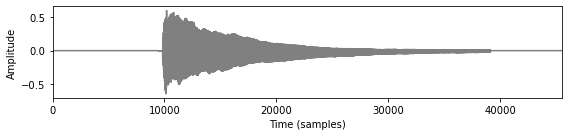MP3 file:  Fs = 11025, x.shape = (47232,), x.dtype = float32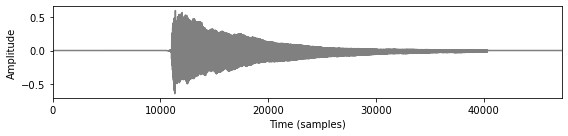## PySoundFile¶

The audio library PySoundFile yields functions for reading and writing sound files. In particular, it contains the functions soundfile.read and soundfile.write.

• Per default, the loaded audio is converted to a float with amplitude values lying in the range of $[-1, 1]$. This default can be changed using the dtype keyword.
• When writing, it uses signed $16$-bit PCM (subtype='PCM_16') as default.
• There are no resampling options.
• There is no option to read MP3-files.
In :
import soundfile as sf

fn_wav = os.path.join('..', 'data', 'B', 'FMP_B_Note-C4_Piano.wav')
print_plot_play(x=x,Fs=Fs,text='WAV file (default): ')

# Read wav with dtype= 'int16'
fn_wav = os.path.join('..', 'data', 'B', 'FMP_B_Note-C4_Piano.wav')
x, Fs = sf.read(fn_wav, dtype= 'int16')
print_plot_play(x=x,Fs=Fs,text='WAV file (dtype=int16): ')

# Write 'int16'-signal and read with default
fn_out = os.path.join('..', 'output', 'B', 'FMP_B_Note-C4_Piano_int16.wav')
sf.write(fn_out, x, Fs)
print_plot_play(x=x,Fs=Fs,text='Signal (int16) after writing and reading (default): ')

WAV file (default):  Fs = 11025, x.shape = (45504,), x.dtype = float64WAV file (dtype=int16):  Fs = 11025, x.shape = (45504,), x.dtype = int16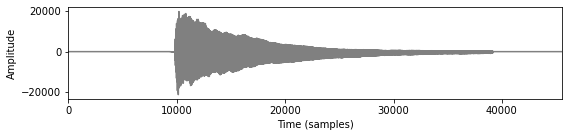Signal (int16) after writing and reading (default):  Fs = 11025, x.shape = (45504,), x.dtype = float64In :
# Generate signal
Fs = 8000
x = 0.5 * np.cos(2 * np.pi * 440 * np.arange(0, Fs) / Fs)
x[2000:2200] = 2
print_plot_play(x=x,Fs=Fs,text='Generated signal: ')

# Write signal
# Default: 'PCM_16'
# Equivalent to pre-processing (dithering + quantization)
# x = np.int16(np.round(x*(2**15)))
#
print('Default for writing files:', sf.default_subtype('WAV'))
fn_out = os.path.join('..', 'output', 'B', 'FMP_B_PythonAudio_sine.wav')
sf.write(fn_out, x, Fs, subtype='PCM_16')

print_plot_play(x=x,Fs=Fs,text='Signal after writing and reading: ')

Generated signal:  Fs = 8000, x.shape = (8000,), x.dtype = float64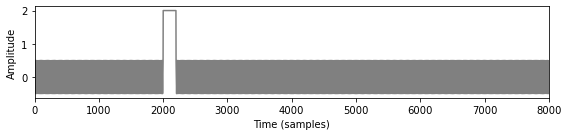Default for writing files: PCM_16
Signal after writing and reading:  Fs = 8000, x.shape = (8000,), x.dtype = float64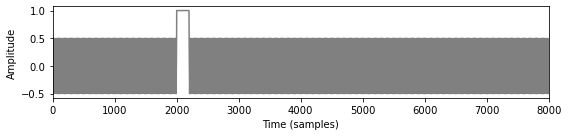## SciPy¶

Scipy offers the scipy.io.wavfile module, which also has functionalities for reading and writing wav files. However, not all variants of the wav format are support. For example, $24$-bit integer WAV-files are not allowed. Furthermore, certain metadata fields in a wav file may also lead to errors. Therefore, we do not recommend this option.

In :
from scipy.io import wavfile


Signal after writing and reading:  Fs = 11025, x.shape = (45504,), x.dtype = int16